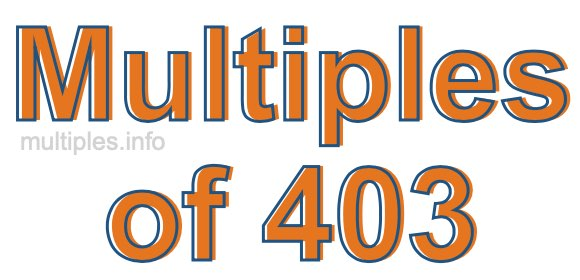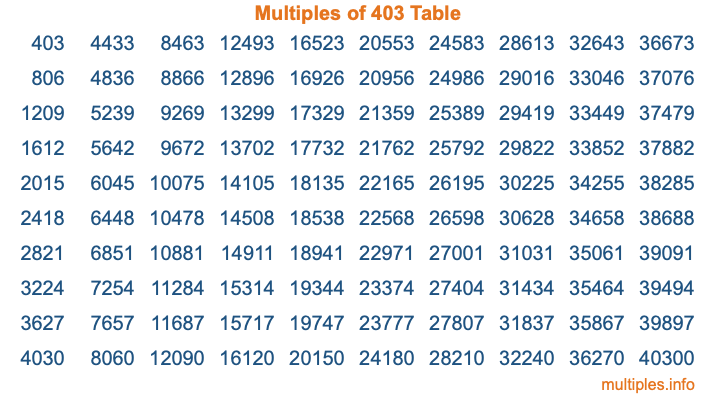Multiples of 403Welcome to the Multiples of 403 page. Here we will first teach you everything you will ever need to know about the multiples of 403, and then give you a study guide summary of everything we taught you to make sure you remember it all. Use this page to look up facts and learn information about the multiples of 403. This page will make you a multiples of four hundred three expert!

Definition of Multiples of 403
Multiples of 403 are all the numbers that when divided by 403 equal an integer. Each of the multiples of 403 are called a multiple. A multiple of 403 is created by multiplying 403 by an integer.

Therefore, to create a list of multiples of 403, you start with 1 multiplied by 403, then 2 multiplied by 403, then 3 multiplied by 403, and so on for as long as you want. Thus, the list of the first five multiples of 403 is 403, 806, 1209, 1612, and 2015. To see a larger list of multiples of 403, see the printable image of Multiples of 403 further down on this page. We also have a category where you can choose any nth multiple of 403.

Multiples of 403 Checker
The Multiples of 403 Checker below checks to see if any number of your choice is a multiple of 403. In other words, it checks to see if there is any number (integer) that when multiplied by 403 will equal your number. To do that, we divide your number by 403. If the the quotient is an integer, then your number is a multiple of 403.

Is  a multiple of 403?

Least Common Multiple of 403 and ...
A Least Common Multiple (LCM) is the lowest multiple that two or more numbers have in common. This is also called the smallest common multiple or lowest common multiple and is useful to know when you are adding our subtracting fractions. Enter one or more numbers below (403 is already entered) to find the LCM.

Check out our LCM Calculator if you need more details about the Least Common Multiple or if you need the LCM for different numbers for adding and subtraction fractions.

nth Multiple of 403
As we stated above, 403 is the first multiple of 403, 806 is the second multiple of 403, 1209 is the third multiple of 403, and so on. Enter a number below to find the nth multiple of 403.

th multiple of 403

Multiples of 403 vs Factors of 403
403 is a multiple of 403 and a factor of 403, but that is where the similarities end. All postive multiples of 403 are 403 or greater than 403. All positive factors of 403 are 403 or less than 403.

Below is the beginning list of multiples of 403 and the factors of 403 so you can compare:

Multiples of 403: 403, 806, 1209, 1612, 2015, etc.

Factors of 403: 1, 13, 31, 403

As you can see, the multiples of 403 are all the numbers that you can divide by 403 to get a whole number. The factors of 403, on the other hand, are all the whole numbers that you can multiply by another whole number to get 403.

It's also interesting to note that if a number (x) is a factor of 403, then 403 will also be a multiple of that number (x).

Multiples of 403 vs Divisors of 403
The divisors of 403 are all the integers that 403 can be divided by evenly. Below is a list of the divisors of 403.

Divisors of 403: 1, 13, 31, 403

The interesting thing to note here is that if you take any multiple of 403 and divide it by a divisor of 403, you will see that the quotient is an integer.

Multiples of 403 Table
Below is an image of the first 100 multiples of 403 in a table. The table is in chronological order, column by column. The first column has the first ten multiples of 403, the second column has the next ten multiples of 403, and so on.The Multiples of 403 Table is also referred to as the 403 Times Table or Times Table of 403. You are welcome to print out our table for your studies.

Negative Multiples of 403
Although not often discussed or needed in math, it is worth mentioning that you can make a list of negative multiples of 403 by multiplying 403 by -1, then by -2, then by -3, and so on, to get the following list of negative multiples of 403:

-403, -806, -1209, -1612, -2015, etc.

Multiples of 403 Summary
Below is a summary of important Multiples of 403 facts that we have discussed on this page. To retain the knowledge on this page, we recommend that you read through the summary and explain to yourself or a study partner why they hold true.

There are an infinite number of multiples of 403.

A multiple of 403 divided by 403 will equal a whole number.

403 divided by a factor of 403 equals a divisor of 403.

The nth multiple of 403 is n times 403.

The largest factor of 403 is equal to the first positive multiple of 403.

403 is a multiple of every factor of 403.

403 is a multiple of 403.

A multiple of 403 divided by a divisor of 403 equals an integer.

403 divided by a divisor of 403 equals a factor of 403.

Any integer times 403 will equal a multiple of 403.

Multiples of a Number
Here you can get the multiples of another number, all with the same attention to detail as we did for multiples of 403 on this page.

Multiples of
Multiples of 404
Did you find our page about multiples of four hundred three educational? Do you want more knowledge? Check out the multiples of the next number on our list!